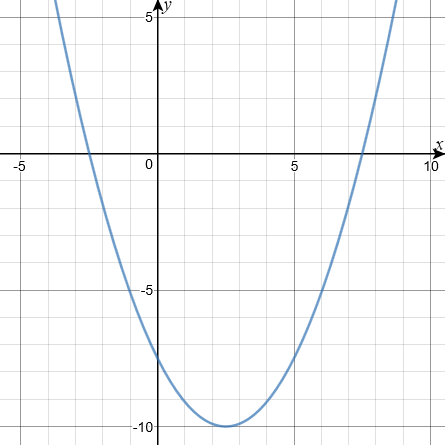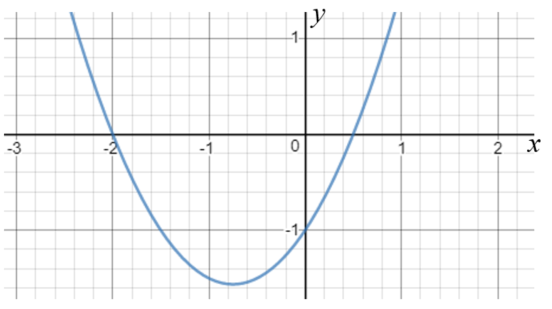# Exam-Style Questions.

## Problems adapted from questions set for previous Mathematics exams.

### 1.

GCSE Higher

(a) Solve 9m < 15m − 12

(b) On the number line below, show the set of values of $$x$$ for which $$-4 \le x-1 \lt 5$$### 2.

GCSE Higher

The graph of a quadratic function, $$y=f(x)$$ is shown drawn accurately in the following diagram. Write down all the integer solutions of $$f(x) \le 0$$.### 3.

GCSE Higher

The diagram below is a sketch of $$y = f(x)$$ where $$f(x)$$ is a quadratic function.

The graph intersects the x-axis where $$x=-2$$ and $$x = 0.5$$.Which of the following is the solution of $$f(x) \le 0$$ ?

• (a) $$x \ge -2$$ or $$x \ge 0.5$$

• (b) $$-2 \ge x \ge 0.5$$

• (c) $$x \ge -2$$ and $$x \le 0.5$$

• (d) $$x \le -2$$ and $$x \ge 0.5$$

### 4.

GCSE Higher

Describe the unshaded (white) region by writing down three inequalities.### 5.

GCSE Higher

On the grid below indicate the region that satisfies all three of these inequalities.

$$y>-2$$ $$x+y<5$$ $$y-1 \le \frac{x}{2}$$### 6.

GCSE Higher

A region on a coordinate grid is described by the following three inequalities:

$$x>-2$$ $$x+y<7$$ $$y \ge \frac{x}{3}+2$$

By shading the unwanted regions show the region on the grid below.### 7.

GCSE Higher

By shading the unwanted regions, show the region satisfied by these three inequalities.

$$y \le x + 3$$ $$y> 4-x$$ $$x < 2.5$$### 8.

GCSE Higher

Solve the following inequalities then explain how the whole number solutions to A and B different.

$$A: 5 \le 5x \lt 30$$ $$B: 5 \lt 5x \le 30$$

### 9.

GCSE Higher

Show that you understand equations and inequalities by answering the following:

(a) Solve $$5x^2=80$$

(b) Solve $$8x + 2 \gt x + 7$$

(c) Write down the largest integer that satisfies $$8x - 2 \lt 25$$

(d) Solve the following pair of equations

$$3x + 5y = 21$$ $$8x - 5y = 1$$

### 10.

GCSE Higher

Solve $$2x^2 + 7x - 15 <0$$

If you would like space on the right of the question to write out the solution try this Thinning Feature. It will collapse the text into the left half of your screen but large diagrams will remain unchanged.

The exam-style questions appearing on this site are based on those set in previous examinations (or sample assessment papers for future examinations) by the major examination boards. The wording, diagrams and figures used in these questions have been changed from the originals so that students can have fresh, relevant problem solving practice even if they have previously worked through the related exam paper.

The solutions to the questions on this website are only available to those who have a Transum Subscription.

Exam-Style Questions Main Page

Search for exam-style questions containing a particular word or phrase:

To search the entire Transum website use the search box in the grey area below.